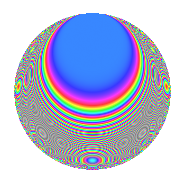# Properties

 Label 504.2.iLevel 504 Weight 2 Character orbit i Rep. character $$\chi_{504}(125,\cdot)$$ Character field $$\Q$$ Dimension 32 Newform subspaces 2 Sturm bound 192 Trace bound 1

# Related objects

## Defining parameters

 Level: $$N$$ = $$504 = 2^{3} \cdot 3^{2} \cdot 7$$ Weight: $$k$$ = $$2$$ Character orbit: $$[\chi]$$ = 504.i (of order $$2$$ and degree $$1$$) Character conductor: $$\operatorname{cond}(\chi)$$ = $$168$$ Character field: $$\Q$$ Newform subspaces: $$2$$ Sturm bound: $$192$$ Trace bound: $$1$$ Distinguishing $$T_p$$: $$5$$

## Dimensions

The following table gives the dimensions of various subspaces of $$M_{2}(504, [\chi])$$.

Total New Old
Modular forms 104 32 72
Cusp forms 88 32 56
Eisenstein series 16 0 16

## Trace form

 $$32q + O(q^{10})$$ $$32q - 4q^{16} + 28q^{22} - 32q^{25} + 4q^{28} - 44q^{46} - 16q^{49} - 4q^{58} + 96q^{64} - 24q^{70} - 16q^{79} - 76q^{88} + O(q^{100})$$

## Decomposition of $$S_{2}^{\mathrm{new}}(504, [\chi])$$ into newform subspaces

Label Dim. $$A$$ Field CM Traces $q$-expansion
$$a_2$$ $$a_3$$ $$a_5$$ $$a_7$$
504.2.i.a $$8$$ $$4.024$$ 8.0.157351936.1 $$\Q(\sqrt{-7})$$ $$0$$ $$0$$ $$0$$ $$0$$ $$q+\beta _{1}q^{2}+\beta _{2}q^{4}+(\beta _{2}-\beta _{5})q^{7}+\beta _{3}q^{8}+\cdots$$
504.2.i.b $$24$$ $$4.024$$ None $$0$$ $$0$$ $$0$$ $$0$$

## Decomposition of $$S_{2}^{\mathrm{old}}(504, [\chi])$$ into lower level spaces

$$S_{2}^{\mathrm{old}}(504, [\chi]) \cong$$ $$S_{2}^{\mathrm{new}}(168, [\chi])$$$$^{\oplus 2}$$